# SQL Server / Query Optimization / String functions in the WHERE clause and bad estimates

Recently we had really nice meetup gathering here in Auckland NZ. Was a beautiful evening, there were 20 people talking about SQL server, was perfect. One of the topics was “String functions in the where clause and bad estimates”

For this demo I used SQL Server 2014 Developer Edition.

As a database I used StackOverflow, you can download this DB from Brent Ozar web page, just follow the instructions

Using string function in the WHERE clause can cause bad estimates.

I will query TAB Badges (has just clustered index on ID column). In the WHERE clause I will use STRING FUNCTION LEFT. LEFT Returns the left part of a character string with the specified number of characters.

```SET STATISTICS TIME, IO ON
DBCC FREEPROCCACHE
SELECT [Name]
,[UserId]
WHERE Left(Name,4) like 'Beta%'
SET STATISTICS IO OFF```

We can see that we have 155202 logical reads, and query returning 2511 rows

(2511 row(s) affected)

Since we do not have Non-Clustered index on that table optimizer use Clustered index to scan whole table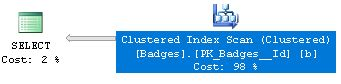If you want to check size of your indexes you can use following query

```USE StackOverflow
SELECT i.[name] AS IndexName, SUM(s.[used_page_count]) * 8 AS IndexSizeKB, SUM(s.[used_page_count]) PageCountNumber
FROM sys.dm_db_partition_stats AS s
INNER JOIN sys.indexes AS i ON s.[object_id] = i.[object_id]
AND s.[index_id] = i.[index_id]
GROUP BY i.[name]
ORDER BY i.[name]
GO```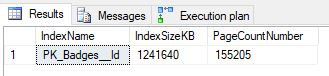Lets now check the estimates for that Clustered Index Scan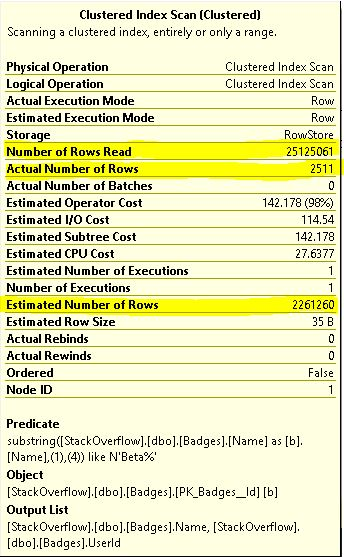For 2511 Actual Number of Rows we have Estimated 2261260, over two million rows, and since we have a scan we are reading every single row in that table 25125061, over 25 million rows

Exact number of rows for Badges table.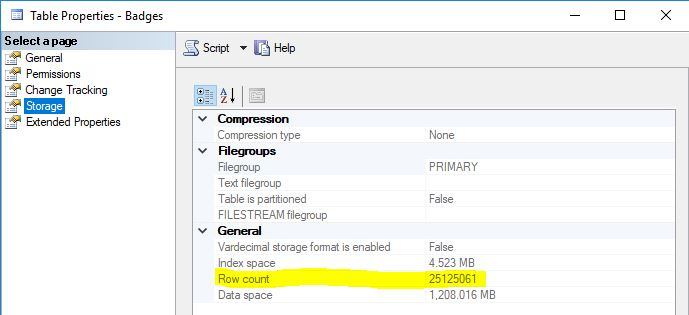Lets try now next query

It is the same query but this time without LEFT function within WHERE clause

```DBCC FREEPROCCACHE
SET STATISTICS TIME, IO ON
SELECT [Name]
,[UserId]
WHERE Name like 'Beta%'
SET STATISTICS IO OFF```

Execution plan is still the same.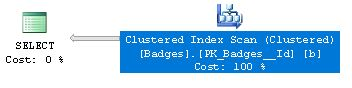But estimates are more accurate now. Estimated number of rows is now 6748, actual number of rows is the same as before as well as number of rows read, since we still do not have non-clustered index on that table.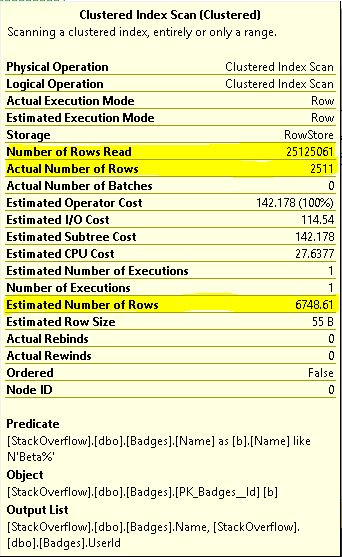If we change our WHERE clause little bit more and use ‘=’ instead of ‘like’ we will have equal number of estimates and actual rows number. Number of rows read stay the same until we cover this query with non-clustered index

```DBCC FREEPROCCACHE
SET STATISTICS TIME, IO ON
SELECT [Name]
,[UserId]
WHERE Name= 'Beta'
SET STATISTICS IO OFF```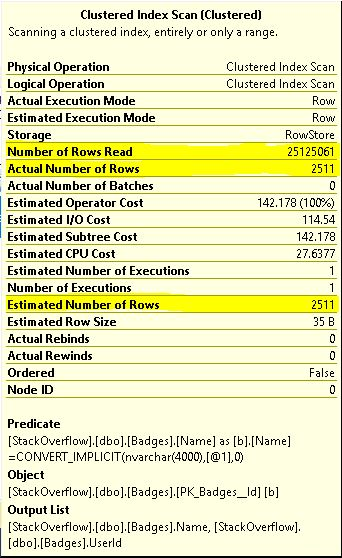```CREATE NONCLUSTERED INDEX [NCI_Name] ON [dbo].[Badges]
(
[Name] ASC,
[Id] ASC
)
INCLUDE ( [UserId]) WITH (PAD_INDEX = OFF, STATISTICS_NORECOMPUTE = OFF, SORT_IN_TEMPDB = OFF, DROP_EXISTING = OFF, ONLINE = OFF, ALLOW_ROW_LOCKS = ON, ALLOW_PAGE_LOCKS = ON)
GO```

If we run the same query again we will have following plan.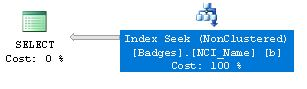We can see that query optimizer using non-clustered index seek to retrieve data from the table and our estimates, actual number of rows and actual number of rows read are the same, 2511.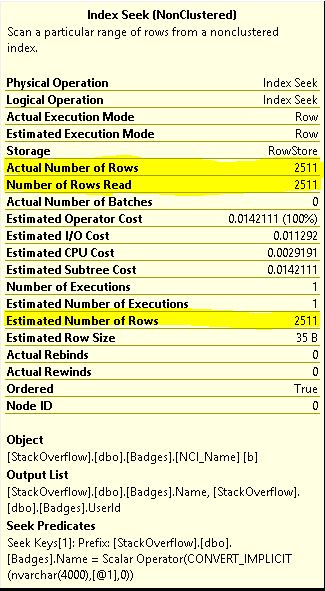Now we have just 15 logical reads, instead of 155202 logical reads like in the first query.

(2511 row(s) affected)

In the following example you can see how the bad estimates can cause excessive memory grant.

### 2 Responses to “ “SQL Server / Query Optimization / String functions in the WHERE clause and bad estimates”

1.Jason says:

with column statistics, Sql server uses column histogram to estimate number of rows

•Barac says:

I agree with you, this was just an example how estimated number of rows can be off with an actual.